# AP Board 8th Class Maths Solutions Chapter 1 Rational Numbers Ex 1.1

AP State Syllabus AP Board 8th Class Maths Solutions Chapter 1 Rational Numbers Ex 1.1 Textbook Questions and Answers.

## AP State Syllabus 8th Class Maths Solutions 1st Lesson Rational Numbers Exercise 1.1Question 1.
Name the properly Involved in the following examples.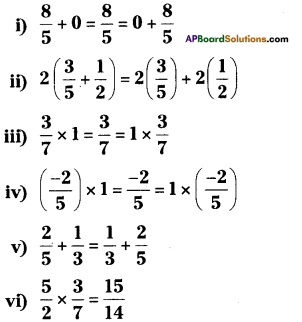vii) 7a + (-7) = 0
viii) x + $$\frac{1}{x}$$ = 1(x ≠ 0)
ix) (2 x x) + (2 x 6) = 2 x (x + 6)
Solution:
ii) Distributive law
iii) Multiplicative identity
iv) Multiplicative identity
vi) Closure law in multiplication
viii) Multiplicative inverse
ix) DistributiveQuestion 2.
Write the additive and the multiplicative inverses of the following.
i) $$\frac{-3}{5}$$
ii) 1
iii) 0
iv) $$\frac{7}{9}$$
v) -1
Solution: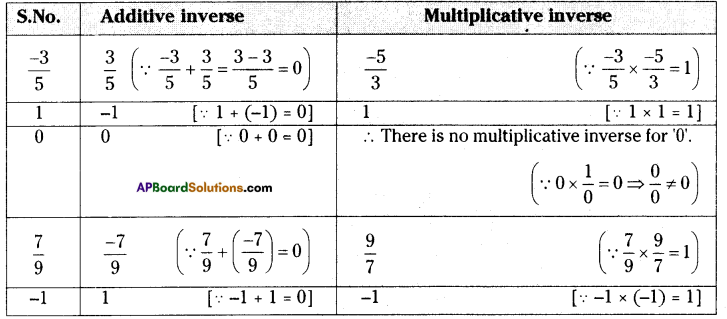Question 3.
Fill in the blanks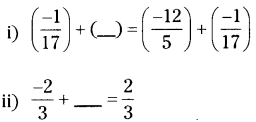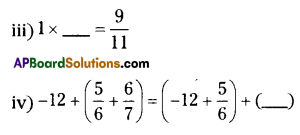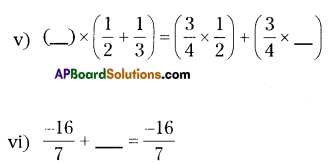Solution:
i) $$\left(\frac{-12}{5}\right)$$
ii) $$\left(\frac{4}{3}\right)$$
iii) $$\left(\frac{9}{11}\right)$$
iv) $$\left(\frac{6}{7}\right)$$
v) $$\left(\frac{3}{4}, \frac{1}{3}\right)$$
vi) 0Question 4.
Multiply $$\frac{2}{11}$$ by the reciprocal of $$\frac{-5}{14}$$
Solution:
The reciprocal of $$\frac{-5}{14}$$ is $$\frac{-14}{5}$$
( ∵ $$\left(\frac{-5}{14}\right) \times\left(\frac{-14}{5}\right)=1$$ )
∴ The product of $$\frac{2}{11}$$ and $$\frac{-14}{5}$$ is
$$\frac{2}{11} \times\left(\frac{-14}{5}\right)=\frac{-28}{55}$$

Question 5.
Which properties can be used computing $$\frac{2}{5} \times\left(5 \times \frac{7}{6}\right)+\frac{1}{3} \times\left(3 \times \frac{4}{11}\right)$$
Solution:
The following properties are involved in the product of
$$\frac{2}{5} \times\left(5 \times \frac{7}{6}\right)+\frac{1}{3} \times\left(3 \times \frac{4}{11}\right)$$
i) Multiplicative associative property.
ii) Multiplicative inverse.
iii) Multiplicative identity.

Question 6.
Verify the following
$$\left(\frac{5}{4}+\frac{-1}{2}\right)+\frac{-3}{2}=\frac{5}{4}+\left(\frac{-1}{2}+\frac{-3}{2}\right)$$
Solution: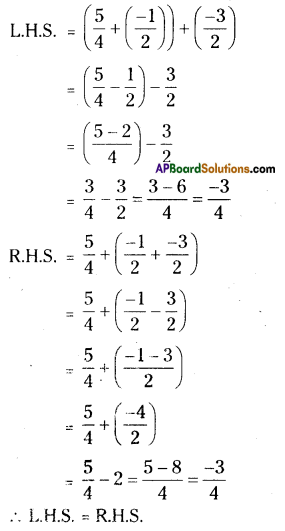Question 7.
Evaluate $$\frac{3}{5}+\frac{7}{3}+\left(\frac{-2}{5}\right)+\left(\frac{-2}{3}\right)$$ after rearrangement.
Solution: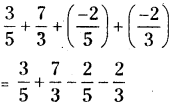Let x = $$1.2 \overline{4}$$
⇒ x = 1.244……. …………………(1)
Here periodicity of equation (1) is 1. So
it should be multiplied by 10 on both
sides.
⇒ 10 x x = 10 x 1.244
10x = 12.44 …………..(2)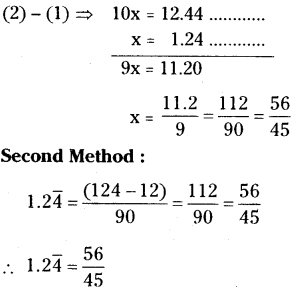Question 8.
Subtract
(i) $$\frac{3}{4}$$ from $$\frac{1}{3}$$
(ii) $$\frac{-32}{13}$$ from 2
(iii) -7 from $$\frac{-4}{7}$$
Solution: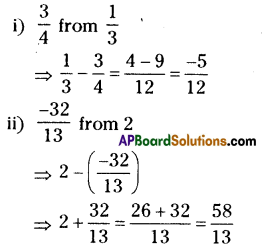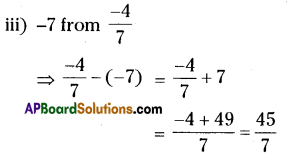Question 9.
What numbers should be added to $$\frac{-5}{8}$$ so as to get $$\frac{-3}{2}$$ ?
Solution:
Let the number to be add ‘x’ say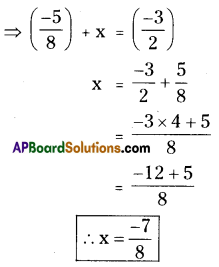∴ $$\frac{-7}{8}$$ should be added to $$\frac{-5}{8}$$ then we will get $$\frac{-3}{2}$$

Question 10.
The sum of two rational numbers is 8 If one of the numbers is $$\frac{-5}{6}$$ find the other.
Let the second number be ‘x’ say
⇒ $$x+\left(\frac{-5}{6}\right)=8$$
⇒$$8+\frac{5}{6}=\frac{48+5}{6}=\frac{53}{6}$$
∴ The other number (x) = $$\frac{53}{6}$$

Question 11.
Is subtraction associative in rational numbers? Explain with an example.
Solution:
Let $$\frac{1}{2}, \frac{3}{4}, \frac{-5}{4}$$ are any 3 rational numbers.
Associative property under subtraction
a – (b – c) = (a – b) – c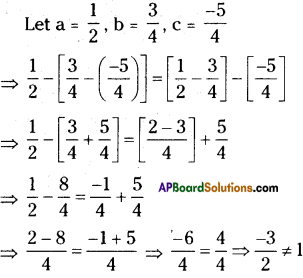∴ L.H.S. ≠ R.H.S.
∴ a – (b – c) ≠ (a – b) – c
∴ Subtraction is not an associative in rational numbers.Question 12.
Verify that – (-x) = x for
(i) x = $$\frac{2}{15}$$
(ii) x = $$\frac{-13}{15}$$
Solution: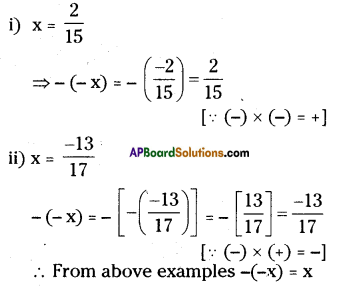Question 13.
Write-
(i) The set of numbers which do not have any additive identity
(ii) The rational number that does not have any reciprocal
(iii) The reciprocal of a negative rational number.
Solution:
i) Set of natural numbers ’N’ doesn’t possesses the number ‘0’.
ii) The rational number ‘0’ has no multiplicative inverse.
[ ∵ 1/0 is not defined]
iii) The reciprocal of a negative rational number is a negative rational number.
Ex : Reciprocal of $$\frac{-2}{5}=\frac{-5}{2}$$